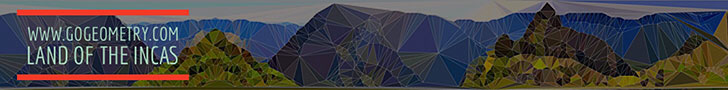Geometry Problems 621-630: Triangle, Circle, Clawson Point, Centers, Similarity# Geometry Problems 621 - 630Geometry Problem 621
Three Rectangles, Diagonals, Centers, Angles.

Geometry Problem 622
Square with Perpendiculars, Transversal, Congruence.

Geometry Problem 623
Triangle, Incenter, Excenter, Incircle, Excircle, Concyclic Points.

Geometry Problem 624
Triangle, Incenter, Excenter, Incircle, Excircle, Similarity.

Geometry Problem 625
Triangle, Parallel Lines, Congruence, Angle Bisector.

Geometry Problem 626
Triangle, Distance from the Incenter to an Excenter.

Geometry Problem 627